prueba

Página de prueba

1.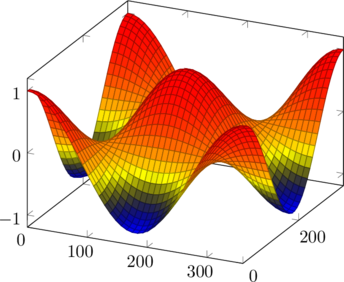2.
At first, we sample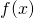in the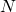(is odd) equidistant points around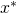: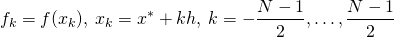where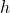is some step.
Then we interpolate points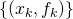by polynomial

(1)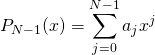Its coefficients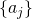are found as a solution of system of linear equations:

(2)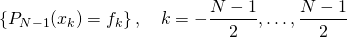Here are references to existing equations: (1), (2).
Here is reference to non-existing equation (??).